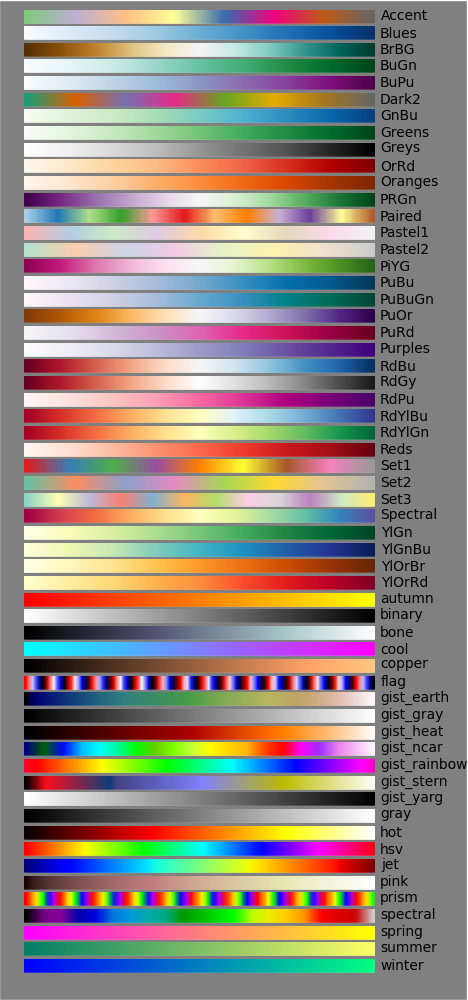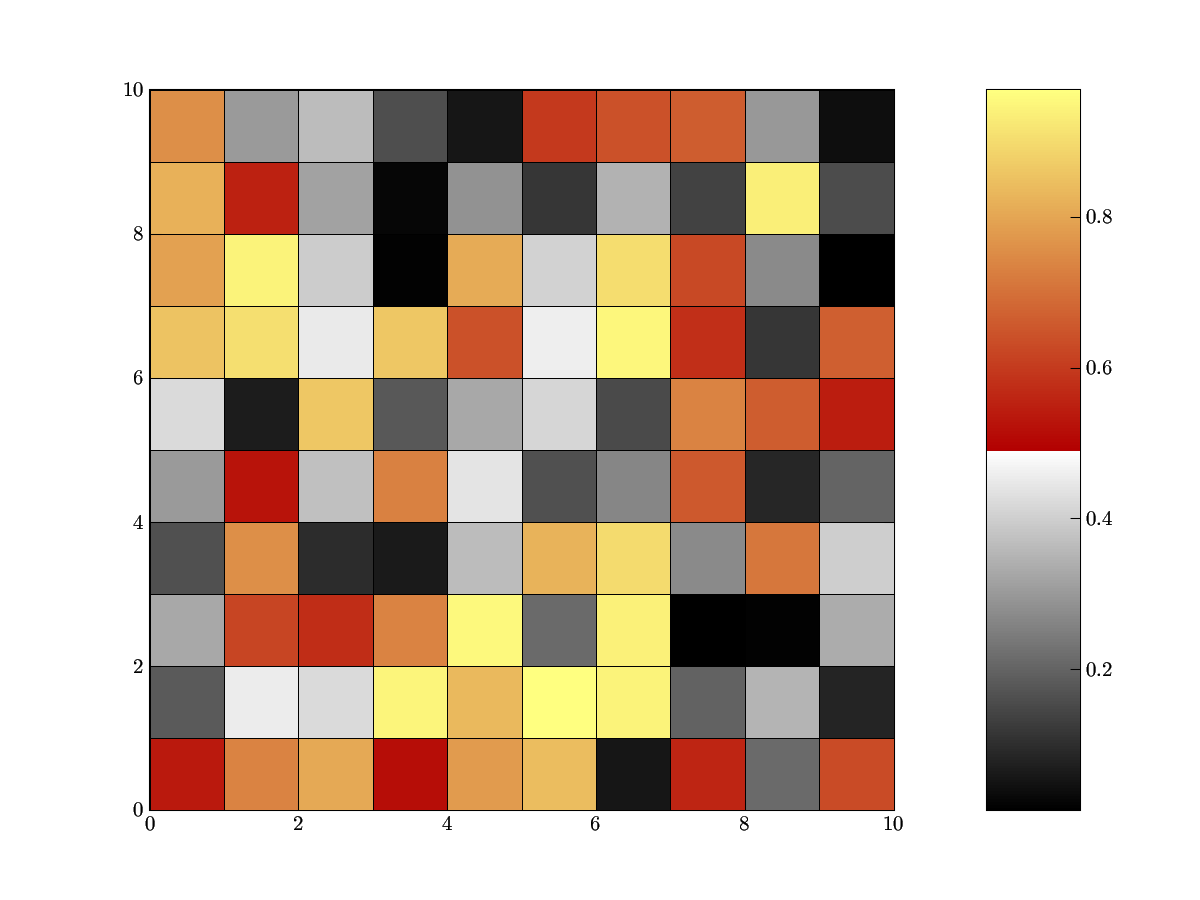# Matplotlib: show colormaps¶

Show Matplotlib colormaps

In [ ]:
#!python
from pylab import *
from numpy import outer
rc('text', usetex=False)
a=outer(arange(0,1,0.01),ones(10))
figure(figsize=(10,5))
maps=[m for m in cm.datad if not m.endswith("_r")]
maps.sort()
l=len(maps)+1
for i, m in enumerate(maps):
subplot(1,l,i+1)
axis("off")
imshow(a,aspect='auto',cmap=get_cmap(m),origin="lower")
title(m,rotation=90,fontsize=10)
savefig("colormaps.png",dpi=100,facecolor='gray')But, what if I think those colormaps are ugly? Well, just make your own using matplotlib.colors.!LinearSegmentedColormap.

First, create a script that will map the range (0,1) to values in the RGB spectrum. In this dictionary, you will have a series of tuples for each color 'red', 'green', and 'blue'. The first elements in each of these color series needs to be ordered from 0 to 1, with arbitrary spacing inbetween. Now, consider (0.5, 1.0, 0.7) in the 'red' series below. This tuple says that at 0.5 in the range from (0,1) , interpolate from below to 1.0, and above from 0.7. Often, the second two values in each tuple will be the same, but using diferent values is helpful for putting breaks in your colormap. This is easier understand than might sound, as demonstrated by this simple script:

In [ ]:
#!python
from pylab import *
cdict = {'red': ((0.0, 0.0, 0.0),
(0.5, 1.0, 0.7),
(1.0, 1.0, 1.0)),
'green': ((0.0, 0.0, 0.0),
(0.5, 1.0, 0.0),
(1.0, 1.0, 1.0)),
'blue': ((0.0, 0.0, 0.0),
(0.5, 1.0, 0.0),
(1.0, 0.5, 1.0))}
my_cmap = matplotlib.colors.LinearSegmentedColormap('my_colormap',cdict,256)
pcolor(rand(10,10),cmap=my_cmap)
colorbar()As you see, the colormap has a break halfway through. Please use this new power responsibly.

Here a slightly modified version of the above code which allows for displaying a selection of the pre-defined colormaps as well as self-created registered colormaps. Note that the cmap_d dictionary in the cm module is not documented. The choice of indexed colors in discrete_cmap is somewhat haphazardous...

In [ ]:
"""Python colormaps demo

includes:
examples for registering own color maps
utility for showing all or selected named colormaps including self-defined ones"""

import matplotlib
import matplotlib.colors as col
import matplotlib.cm as cm
import matplotlib.pyplot as plt
import numpy as np

def register_own_cmaps():
"""define two example colormaps as segmented lists and register them"""
# a good guide for choosing colors is provided at
# http://geography.uoregon.edu/datagraphics/color_scales.htm
#
# example 1:
# create own colormap from purple, blue, green, orange to red
# cdict contains a tuple structure for 'red', 'green', and 'blue'.
# Each color has a list of (x,y0,y1) tuples, where
# x defines the "index" in the colormap (range 0..1), y0 is the
# color value (0..1) left of x, and y1 the color value right of x.
# The LinearSegmentedColormap method will linearly interpolate between
# (x[i],y1) and (x[i+1],y0)
# The gamma value denotes a "gamma curve" value which adjusts the brightness
# at the bottom and top of the colormap. According to matlab documentation
# this means:
# colormap values are modified as c^gamma, where gamma is (1-beta) for
# beta>0 and 1/(1+beta) for beta<=0
cdict = {'red': ((0.0, 0.0, 0.0),
(0.3, 0.5, 0.5),
(0.6, 0.7, 0.7),
(0.9, 0.8, 0.8),
(1.0, 0.8, 0.8)),
'green': ((0.0, 0.0, 0.0),
(0.3, 0.8, 0.8),
(0.6, 0.7, 0.7),
(0.9, 0.0, 0.0),
(1.0, 0.7, 0.7)),
'blue': ((0.0, 1.0, 1.0),
(0.3, 1.0, 1.0),
(0.6, 0.0, 0.0),
(0.9, 0.0, 0.0),
(1.0, 1.0, 1.0))}
cmap1 = col.LinearSegmentedColormap('my_colormap',cdict,N=256,gamma=0.75)
cm.register_cmap(name='own1', cmap=cmap1)

# example 2: use the "fromList() method
startcolor = '#586323'  # a dark olive
midcolor = '#fcffc9'    # a bright yellow
endcolor = '#bd2309'    # medium dark red
cmap2 = col.LinearSegmentedColormap.from_list('own2',[startcolor,midcolor,endcolor])
# extra arguments are N=256, gamma=1.0
cm.register_cmap(cmap=cmap2)
# we can skip name here as it was already defined

def discrete_cmap(N=8):
"""create a colormap with N (N<15) discrete colors and register it"""
# define individual colors as hex values
cpool = [ '#bd2309', '#bbb12d', '#1480fa', '#14fa2f', '#000000',
'#faf214', '#2edfea', '#ea2ec4', '#ea2e40', '#cdcdcd',
'#577a4d', '#2e46c0', '#f59422', '#219774', '#8086d9' ]
cmap3 = col.ListedColormap(cpool[0:N], 'indexed')
cm.register_cmap(cmap=cmap3)

def show_cmaps(names=None):
"""display all colormaps included in the names list. If names is None, all
defined colormaps will be shown."""
# base code from http://www.scipy.org/Cookbook/Matplotlib/Show_colormaps
matplotlib.rc('text', usetex=False)
a=np.outer(np.arange(0,1,0.01),np.ones(10))   # pseudo image data
f=plt.figure(figsize=(10,5))
# get list of all colormap names
# this only obtains names of built-in colormaps:
maps=[m for m in cm.datad if not m.endswith("_r")]
# use undocumented cmap_d dictionary instead
maps = [m for m in cm.cmap_d if not m.endswith("_r")]
maps.sort()
# determine number of subplots to make
l=len(maps)+1
if names is not None: l=len(names)  # assume all names are correct!
# loop over maps and plot the selected ones
i=0
for m in maps:
if names is None or m in names:
i+=1
ax = plt.subplot(1,l,i)
ax.axis("off")
plt.imshow(a,aspect='auto',cmap=cm.get_cmap(m),origin="lower")
plt.title(m,rotation=90,fontsize=10,verticalalignment='bottom')
plt.savefig("colormaps.png",dpi=100,facecolor='gray')

if __name__ == "__main__":
register_own_cmaps()
discrete_cmap(8)
show_cmaps(['indexed','Blues','OrRd','PiYG','PuOr',
'RdYlBu','RdYlGn','afmhot','binary','copper',
'gist_ncar','gist_rainbow','own1','own2'])


Section author: AndrewStraw, Unknown, Unknown, NeilMB, Unknown, DavidLinke, Unknown, newacct, Unknown

Attachments